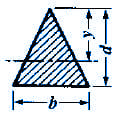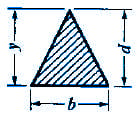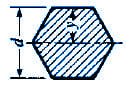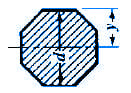Moment of Inertia, Section Modulus, Radii of Gyration Equations
Triangular, Hex Sections

Moment of Inertia, Section Modulus, Radii of Gyration Equations Triangular, Hex Sections

 A = Area (in2, mm2) I = Moment of Inertia (in4, mm4) Gr = Radius of Gyration = (in, mm)or (I/A)1/2 y = Distance of Axis to Extreme Fiber (in, mm)

 SectionOpen Section Properties Case 11 CalculatorOpen Section Properties Case 12 CalculatorOpen Section Properties Case 13 Calculator Moment of InertiaRadius of GyrationAreaySectionOpen Section Properties Case 14 CalculatorOpen Section Properties Case 15 Calculator Moment of InertiaRadius of GyrationAreaySectionOpen Section Properties Case 16 Calculator Moment of InertiaRadius of GyrationAreayRelated:Membership Register | LoginHomeEngineering Book StoreEngineering ForumExcel App. DownloadsOnline Books & ManualsEngineering NewsEngineering VideosEngineering CalculatorsEngineering ToolboxGD&T Training Geometric Dimensioning TolerancingDFM DFA TrainingTraining Online EngineeringAdvertising CenterCopyright Notice